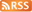•加入收藏  设为首页

# 利用挂机打元宝功能实现自动存取极品装备脚本示例

aa=取背包中物品数量("骑士手镯")
for sa=1,aa do

end
aa=取背包中物品数量("力量戒指")
for sa=1,aa do

end
aa=取背包中物品数量("龙之手镯")
for sa=1,aa do

end
aa=取背包中物品数量("紫碧螺")
for sa=1,aa do

end
aa=取背包中物品数量("泰坦戒指")
for sa=1,aa do

end
aa=取背包中物品数量("三眼手镯")
for sa=1,aa do

end

致力打造绿色安全玩家交流网